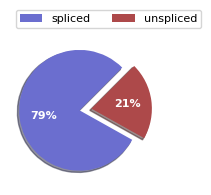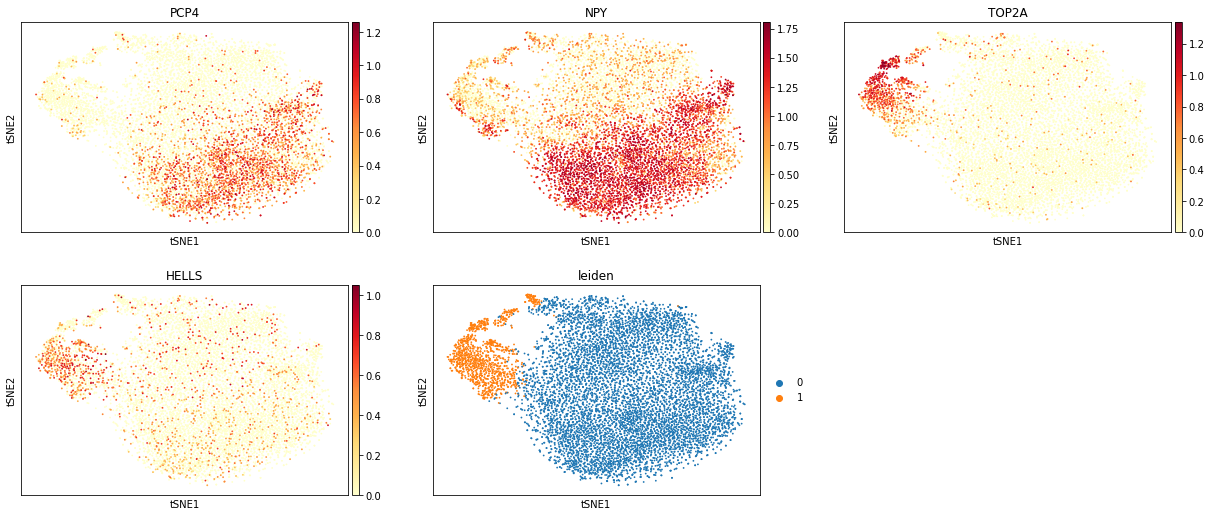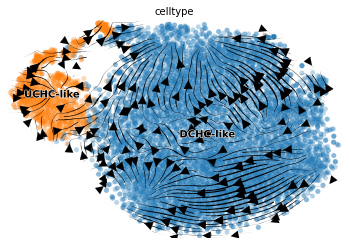In this notebook, we will use scVelo (Bergen et al.) to do RNA-velocity analysis for a primary tumor sample of patient T71 from the dataset GSE137804 (Transcriptomic Characterization of neuroblastoma by Single Cell RNA Sequencing, Donr R. 2020)

After projecting the primary tumor cells from neuroblastoma patients into a reference atlas of early human embryos and fetal adrenal glands at relatively late development stages, the authors found that tumor cells from patient data resemble two sub-populations: cycling chromaffin cells as undifferentiated chromaffin cells (UCHCs) and non-cycling chromaffin cells as differentiated chromaffin cells (DCHCs).

We will begin with identifying two sub-populations of tumor cells in this sample, and use scVelo to predict the progression from one tumor sub-population to another.

In :
import scvelo as scv
import scanpy as sc
import urllib.request


The input of this notebook is an Adata object generated by the Kallisto BUS velocity pipeline. We intersected the cell barcodes generated by Kallisto with the filtered cells annotation provided by the author to produce an AnnData object of 9398 cells, whic are tumor cells only. The AnnData object has two layers of expression data which are spliced and unspliced

In :
patient_t71, _ = urllib.request.urlretrieve(
)

In :
adata = sc.read_h5ad('GSE137804-kallisto.symbol.h5ad')

Out:
AnnData object with n_obs × n_vars = 9398 × 58367
obs: 'sample', 'celltype'
layers: 'spliced', 'unspliced'

Display the proportion of spliced molecules and unspliced molecules in this dataset

In :
scv.pl.proportions(adata)Depending on the protocol used (Drop-Seq, Smart-Seq), there are about 10%-25% of unspliced mRNA molecules which containing intronic sequences.

### Basic preprocessing the data¶

Preprocessing requisites consist of gene selection by detection (with a minimum number of counts) and high variability (dispersion), normalizing every cell by its total size and logarithmizing X. Filtering and normalization is applied in the same vein to spliced/unspliced counts and X. Logarithmizing is only applied to X. If X is already preprocessed from former analysis, it will not be touched.

In :
scv.pp.filter_and_normalize(adata, min_shared_counts=3, n_top_genes=4000)

Filtered out 48829 genes that are detected 3 counts (shared).
Normalized count data: X, spliced, unspliced.
Extracted 4000 highly variable genes.
Logarithmized X.


Computes moments for velocity estimation. First-/second-order moments are computed for each cell across its nearest neighbors, where the neighbor graph is obtained from euclidean distances in PCA space.

In :
scv.pp.moments(adata, n_pcs=30, n_neighbors=30)

computing neighbors
computing moments based on connectivities
'Ms' and 'Mu', moments of un/spliced abundances (adata.layers)


Compute the t-SNE

In :
sc.tl.tsne(adata)


Do Leiden cell clustering. We suspect that there are two sub-population of tumor cells in this dataset so we use a small resolution

In :
sc.tl.leiden(adata, resolution = 0.2)


We plot the t-SNE with cells colored by some marker genes like PCP4 (chromaffin cell-like marker), TOP2A, and HELLS (cell-cycle markers), and the leiden clustering

In :
sc.pl.tsne(adata, color=['PCP4', 'NPY', 'TOP2A', 'HELLS', 'leiden'], ncols=3, color_map='YlOrRd')Since the author didn't provide the exact annotation for each cell, we will use the marker genes reported in the publication to identify cluster 0 as the DCHC-like cell population, and cluster 1 as the UCHC-like population

In :
adata.obs['celltype'] = (
'0': 'DCHC-like',
'1': 'UCHC-like'
}.get(x, x)).astype('category')
)


### Estimate RNA velocity¶

Velocities are vectors in gene expression space and represent the direction and speed of movement of the individual cells. The velocities are obtained by modeling transcriptional dynamics of splicing kinetics, either stochastically (default) or deterministically (by setting mode='deterministic').

For each gene, a steady-state-ratio of pre-mature (unspliced) and mature (spliced) mRNA counts is fitted, which constitutes a constant transcriptional state. Velocities are then obtained as residuals from this ratio.

In :
scv.tl.velocity(adata)

computing velocities
'velocity', velocity vectors for each individual cell (adata.layers)


The computed velocities are stored in adata.layers just like the count matrices

In :
adata.layers['velocity']

Out:
array([[-0.00843609, -0.01818206, -0.00271271, ..., -0.00329949,
0.12779334,  0.02179979],
[ 0.        , -0.03086859, -0.00939301, ...,  0.        ,
0.14171043,  0.        ],
[ 0.        ,  0.        , -0.01660287, ..., -0.00402984,
-0.03750505,  0.03165812],
...,
[-0.03606855,  0.        ,  0.00575436, ..., -0.003869  ,
-0.00928451,  0.05416595],
[-0.0017605 ,  0.02446539, -0.00124819, ..., -0.00184293,
0.04092183,  0.01954305],
[-0.00179475,  0.00253087, -0.00587782, ..., -0.00213099,
0.14145498,  0.01485818]], dtype=float32)

Positive velocity indicates that a gene is actively being transcribed, which occurs for cells that show higher abundance of unspliced mRNA for that gene than expected in steady state. Conversely, negative velocity indicates that a gene is down-regulated.

In :
scv.tl.velocity_graph(adata)

computing velocity graph (using 1/192 cores)

  0%|          | 0/9398 [00:00<?, ?cells/s]
    finished (0:00:08) --> added
'velocity_graph', sparse matrix with cosine correlations (adata.uns)


### Project the velocities¶

In :
scv.pl.velocity_embedding_stream(adata, basis='tsne', color='celltype', arrow_size=2)

computing velocity embeddingscv.pl.velocity(adata, ['PCP4', 'TOP2A', 'HELLS'], color = 'celltype')